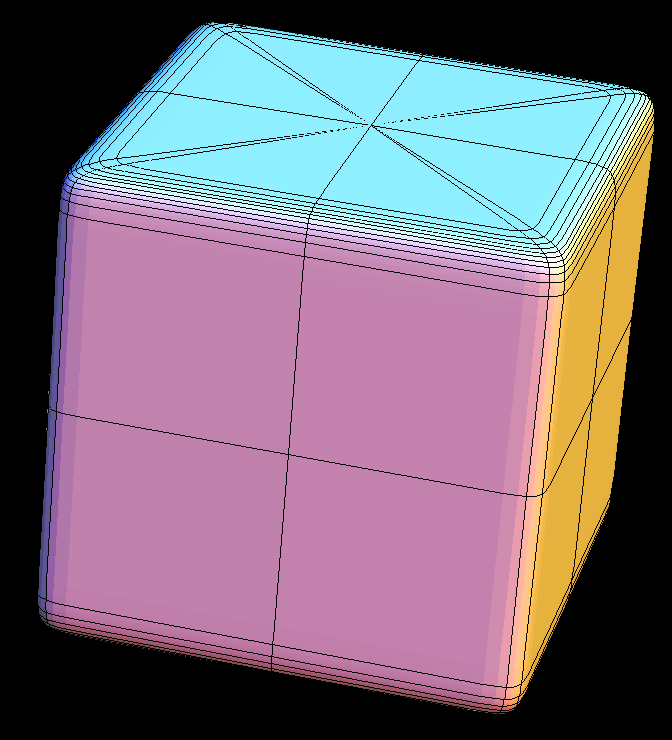# Norm One FamilyThe surfaces are given by the equation |x|^p + |y|^p + |z|^p = 1, where p is a parameter. For p between 1 (octahehedron) and infinity (cube) the surfaces are convex. They are the unit spheres of different Norms for R^3.

Get red/blue stereo glasses from amazon

PlayWithFormulas.pdf